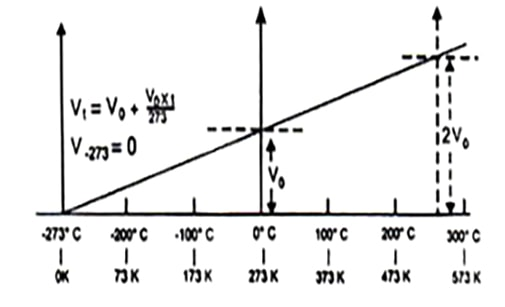Chemistry

# Absolute Zero Temperature

Absolute Zero Temperature:

According to Charles law (at constant pressure) the temperature at which the volume of all gases becomes theoretically zero is known as absolute zero temperature. A temperature below this can not be conceived off in the universe.

Significance of absolute zero temperature:

A temperature below this cannot be conceived in the universe. It is the temperature at which the kinetic energy of atoms and molecules is minimum. At absolute temperature, the movement of molecules is stopped and consequently their average kinetic energy would be zero. It is important to note that this temperature is only hypothetical and cannot be achieved in practice.

Establishment of the scale Absolute of temperature:

This absolute zero temperature provides the starting point for a scale of temperature is known as absolute temperature scale. The absolute scale is known as Kelvin scale at present. It is a thermometric scale where -273°C is the starting point i.e. on this scale 0 K is equal to -273°C and each small division of the scale of absolute temperature is equal to the small division of the centigrade (at present Celsius) scale. The absolute scale starts at -273° of the Celsius scale. The (°C) degree sign is not used while expressing the temperatures on Kelvin scale. Thus,

-273.15°C=0 K
0°C=273 K
1°C= (273+1) K=274 K
100°C= (273+100) K=373 K
t°C= (273+t) K

Thus temperature on the Kelvin scale can be obtained by adding 273.15 to the temperature on Celsius scale.